# ONNX to TF-Lite Model Conversion¶

This tutorial describes how to convert an ONNX formatted model file into a format that can execute on an embedded device using Tensorflow-Lite Micro.

## Overview¶

ONNX is an open data format built to represent machine learning models. Many machine learning frameworks allow for exporting their trained models to this format.
Using the process defined in this tutorial, a machine learning model in the ONNX can be converted to a int8 quantized Tensorflow-Lite format which can be executed on an embedded device.

The basic sequence for this is shown in the following diagram:Once the `.tflite` model is generated, the MLTK’s Model Profiler is used to profile the model to ensure it can efficiently run on an embedded target.

This tutorial describes how to take a model trained by Matlab and run it on an embedded device with Tensorflow-Lite Micro.

The model is a simple CNN and uses the CIFAR10 dataset.

The Matlab model training scripts and trained model may be downloaded from here: cifar10_matlab_model.zip.

NOTE: While the Matlab scripts are provided as a reference, they are out-of-scope for this tutorial.
The key part of the script is the line:

```exportONNXNetwork(trainedNet,'cifar10_matlab_model.onnx')
```

Which converts the trained Matlab model to the ONNX data format. The rest of this tutorial describes how to generate a quantized `.tflite` model file from it.
Other ML frameworks should follow a similar process.

## Considerations¶

Unfortunately, converting from another framework into the Tensorflow-Lite format is not straight-forward. You must be mindful of several aspects when doing the conversion:

### Input Data Format¶

Any preprocessing that is done to the input samples during training must also be done at runtime on the embedded device.
So, for instance, if your training scripts scale the input image by 255 then the images must also be scaled on the embedded device.
Any divergence will cause the ML model to “see” different data and likely reduce accuracy.

### Output Class ID Mapping¶

If the ML model is a classifier, then each class label has a corresponding ID associated with it.
For instance:

Class Label

ID

Left

0

Right

1

Up

2

Down

3

When the model makes a prediction, it returns a probability vector with the index of the largest vector entry mapping to the corresponding predicted class ID.
The mapping used by training must match the mapping used at runtime by the embedded device.

Do not make assumptions about the ID mapping used during training. Frameworks like Matlab use categorical arrays where the entries are not necessarily ordered.

### Supported ML Kernel Operations¶

The operations used by your ML model must also be supported by Tensorflow-Lite Micro.

### “Channels-First” vs “Channels-Last”¶

Most frameworks define their kernel tensors to have the following dimensions:

• N - Number of mini-batch samples in the tensor

• H - The height of the tensor

• W - The width of the tensor

• C - The number of channels (aka depth) of the tensor

The most common dimension ordering is:

• Channels-First: NCHW - The channels come before the height and width dimensions

• Channels-Last: NHWC - The channels come after the height and width dimensions

Tensorflow-Lite Micro only supports Channels-Last while the ONNX format requires Channels-First.
Converting from one format to the other is non-trivial. This tutorial describes how to do the conversion.

### Dataset Required for Quantization¶

The dataset used to train the model is also required to generate a quantized model. Recall that the quantized model is what is loaded onto the embedded device.

Refer to the Post Training Quantization guide for more details.

## Running this tutorial from a notebook¶

For documentation purposes, this tutorial was designed to run within a Jupyter Notebook. The notebook can either run locally on your PC or on a remote server like Google Colab.

## Environment Setup¶

Before converting the `.onnx` formatted model to a `.tflite` formatted model file, we need to setup our Python environment:

### Install Python Dependencies¶

Before running the various code snippets in this tutorial, several Python dependencies must first be installed:

```# Install the MLTK (if necessary)
```
```# Install the standard ONNX Python package
# so that we can read the .onnx formatted model file
!pip install onnx onnx_tf
```
```# Install the onnsim Python package
# This can help reduce the complexity of the generated ONNX model file
# https://github.com/daquexian/onnx-simplifier
!pip install onnx-simplifier onnxruntime
```
```# Install the openvino_dev Python package
# This allows for converting the ONNX model to an intermediate
# format so we can then convert it to a TF-Lite model format
# https://docs.openvino.ai/
!pip install openvino_dev
```
```# Install the openvino2tensorflow Python package
# This allows for converting from the openvino format to the .tflite model format
# We primarily need this so we can convert from the NCHW used by .onnx
# to the NHWC used by .tflite
# https://github.com/PINTO0309/openvino2tensorflow
!pip install openvino2tensorflow  tensorflow_datasets
```

First we need to download the trained ML model in ONNX format.
For this tutorial we use the `cifar10_matlab_model.onnx` example.
If you already have a model then you can skip this step.

```from mltk.utils.archive_downloader import download_verify_extract

ONNX_MODEL_ARCHIVE_URL = 'https://www.dropbox.com/s/8m72rmxtznvaki6/cifar10_matlab_model.zip?dl=1'
ONNX_MODEL_ARCHIVE_SHA1 = 'C53827FC8B765183381CDC338AFB88F735479D97'

url=ONNX_MODEL_ARCHIVE_URL,
dest_subdir='datasets/cifar10_matlab_model',
file_hash=ONNX_MODEL_ARCHIVE_SHA1,
show_progress=True,
)

```
```CIFAR10 Matlab model example download directory: C:/Users/reed/.mltk/datasets/cifar10_matlab_model
```

### Configure Paths¶

First, let’s configure the paths used throughout this tutorial.
You can update these paths as necessary for your particular `.onnx` model.

NOTE: You can view the contents of the `.onnx` model file by dragging and dropping onto the webapge: netron.app

```import os
from mltk.utils.path import create_tempdir

# This contains the path to the pre-trained model in ONNX model format
# Update this path to point to your specific model if necessary
ONNX_MODEL_PATH = f'{cifar10_matlab_model_example_dir}/cifar10_matlab_model.onnx'

# This contains the path to our working directory where all
# generated, intermediate files will be stored.
# For this tutorial, we use a temp directory.
# Update as necessary for your setup
WORKING_DIR = create_tempdir('cifar10_matlab_model_onnx_to_tflite')

assert os.path.exists(ONNX_MODEL_PATH), f'The provided ONNX_MODEL_PATH does not exist at: {ONNX_MODEL_PATH}'
os.makedirs(WORKING_DIR, exist_ok=True)

# Use the filename for the model's name
MODEL_NAME = os.path.basename(ONNX_MODEL_PATH)[:-len('.onnx')]

print(f'ONNX_MODEL_PATH = {ONNX_MODEL_PATH}')
print(f'MODEL_NAME = {MODEL_NAME}')
print(f'WORKING_DIR = {WORKING_DIR}')
```
```ONNX_MODEL_PATH = C:/Users/reed/.mltk/datasets/cifar10_matlab_model/cifar10_matlab_model.onnx
MODEL_NAME = cifar10_matlab_model
WORKING_DIR = E:/reed/mltk/cifar10_matlab_model_onnx_to_tflite
```

We need to load the exact dataset used to train the model.
Additionally, we need to preprocess the input samples the same as what was used to train the model.

In this tutorial, the cifar10_matlab_model.zip model was trained by scaling the samples by 1/255.

Additionally, we need to adjust the class ID mapping so that our local dataset matches what was used to train the model. (Matlab orders the class IDs differently than what is specified by the dataset).

```from tensorflow.keras.datasets import cifar10
import numpy as np

# This is the class label order specified by the dataset
# y_test contains a list of integers that correspond to the indices in this class_labels list
#                   0            1           2      3       4      5      6        7       8       9
class_labels = ['airplane', 'automobile', 'bird', 'cat', 'deer', 'dog', 'frog', 'horse', 'ship', 'truck']

# Load the testing subset of the dataset
# We do not need the training subset for this tutorial
# NOTE: The subset is not particularly important, any subset will work
(_, _), (x_test, y_test) = cifar10.load_data()

# Convert the samples to float32 as that's what
# the trained model expects
x_test = x_test.astype('float32')
# Scale the samples by 255 since that's the preprocessing
# used during model training
x_test = x_test/255.

# Matlab uses a different order of class labels compared to the dataset
# Update the mapping to match what the trained model expects
#                         0       1       2          3          4        5       6       7      8       9
mapped_class_labels = ['frog', 'truck', 'deer', 'automobile', 'bird', 'horse', 'ship', 'cat', 'dog', 'airplane']
CLASS_ID_MAPPING = {
0: 9,
1: 3,
2: 4,
3: 7,
4: 2,
5: 8,
6: 0,
7: 5,
8: 6,
9: 1
}

for i, y in enumerate(np.squeeze(y_test, axis=-1)):
y_test[i] = CLASS_ID_MAPPING[y]

print(f'x_test.shape = {x_test.shape}')
print(f'y_test.shape = {y_test.shape}')
```
```x_test.shape = (10000, 32, 32, 3)
y_test.shape = (10000, 1)
```

### Sanity check: Evaluate the ONNX model¶

As a sanity check, load the ONNX model and run each the the `x_test` samples through it and record the model predictions.

Then evaluate the model predictions verus the expected values, `y_test`

```import onnx
from onnx_tf.backend import prepare

tf_rep = prepare(onnx_model)

n_samples = min(len(x_test), 1000) # Let's evaluate up to 1000 samples
n_classes = len(mapped_class_labels)

# Allocate an array to hold the model predictions
y_pred = np.empty((n_samples, n_classes), dtype=np.float32)
print(f'y_pred.shape = {y_pred.shape}')

# The dataset uses the format: NHWC (i.e. channels last)
# However, the ONNX model expects NCHW (i.e. channels first)
# So transpose the x_test data to be in NCHW format
x_test_channels_first = x_test.transpose(0, 3, 1, 2)

# Iterate through each test sample
print(f'Generating model predictions for each test sample using {ONNX_MODEL_PATH}')
print('Be patient, this may take awhile ...')
for i, x in enumerate(x_test_channels_first[:n_samples]):
# Add the N dimension to the individual sample
# e.g. CHW -> NCHW
x = np.expand_dims(x, 0)
# Run inference on the sample
outputs = tf_rep.run(x)
# Save the model prediction
y_pred[i] = outputs

print('done')
```
```c:\Users\reed\workspace\silabs\github_siliconlabs\mltk\.venv\lib\site-packages\tensorflow_addons\utils\ensure_tf_install.py:53: UserWarning: Tensorflow Addons supports using Python ops for all Tensorflow versions above or equal to 2.7.0 and strictly below 2.10.0 (nightly versions are not supported).
The versions of TensorFlow you are currently using is 2.5.3 and is not supported.
Some things might work, some things might not.
If you were to encounter a bug, do not file an issue.
If you want to make sure you're using a tested and supported configuration, either change the TensorFlow version or the TensorFlow Addons's version.
warnings.warn(
```
```y_pred.shape = (1000, 10)
Generating model predictions for each test sample using C:/Users/reed/.mltk/datasets/cifar10_matlab_model/cifar10_matlab_model.onnx
Be patient, this may take awhile ...
done
```
```from mltk.core import ClassifierEvaluationResults

# Use the MLTK to evaluate the ONNX model predictions
results = ClassifierEvaluationResults(
name=MODEL_NAME,
classes = mapped_class_labels
)

print('Evaluating the ONNX model predictions ...')
results.calculate(y_test[:len(y_pred)], y_pred)
print(results.generate_summary())

results.generate_plots()
```
```Evaluating the ONNX model predictions ...
Name: cifar10_matlab_model
Model Type: classification
Overall accuracy: 83.300%
Class accuracies:
- truck = 93.578%
- automobile = 93.258%
- horse = 93.137%
- frog = 91.964%
- ship = 88.679%
- airplane = 81.553%
- bird = 76.000%
- dog = 75.581%
- deer = 75.556%
- cat = 61.165%
Average ROC AUC: 98.287%
Class ROC AUC:
- automobile = 99.648%
- ship = 99.436%
- truck = 99.085%
- horse = 99.073%
- frog = 98.865%
- airplane = 98.176%
- bird = 97.770%
- dog = 97.640%
- deer = 97.507%
- cat = 95.673%
```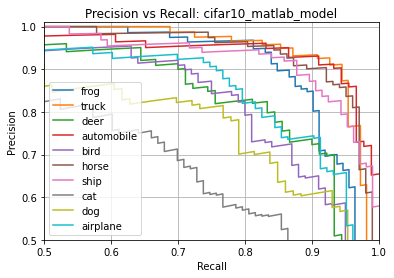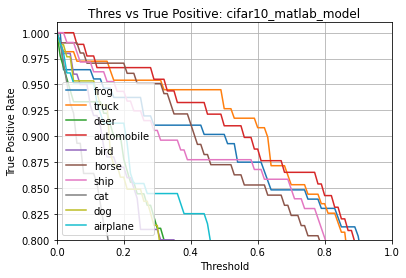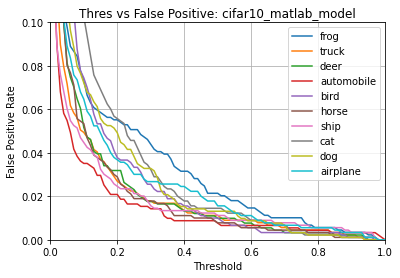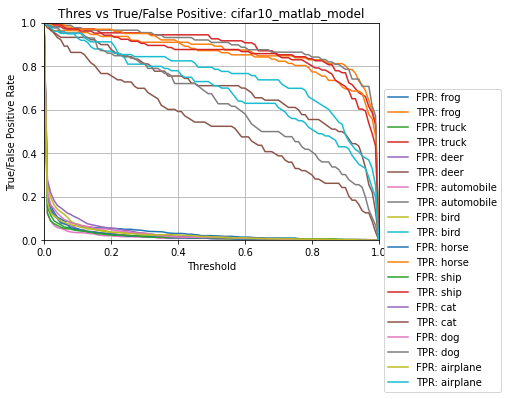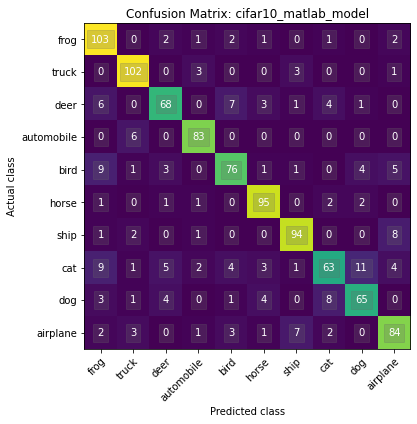## Convert ONNX to Quantized TF-Lite Model File¶

Now that our Python environment is setup and we’re able to get accurate results from our `.onnx` model, we are ready to convert it to a `.tflite` model file.

### Simplify the ONNX model¶

While optional, this step can help reduce the complexity of the ONNX by using the ONNX Simplifier Python package.

This can help reduce the execution overhead on the embedded device.

NOTE: You can view the contents of the generated `.onnx` model file by dragging and dropping onto the webapge: netron.app

```import onnxsim
import onnx

simplified_onnx_model, success = onnxsim.simplify(ONNX_MODEL_PATH)
assert success, 'Failed to simplify the ONNX model. You may have to skip this step'
simplified_onnx_model_path =  f'{WORKING_DIR}/{MODEL_NAME}.simplified.onnx'

print(f'Generating {simplified_onnx_model_path} ...')
onnx.save(simplified_onnx_model, simplified_onnx_model_path)
print('done')
```
```Generating E:/reed/mltk/cifar10_matlab_model_onnx_to_tflite/cifar10_matlab_model.simplified.onnx ...
done
```

### Convert to OpenVino Intermediate Format¶

Recall that the ONNX format uses the `NCHW` format while TF-Lite uses the `NHWC` format to store the model tensors.
While doable, converting from one format to the other is non-trivial. As such, additional steps are required to do the conversion.

The first step is converting the `.onnx` model to the OpenVino intermediate format.
This is done using the tools installed by the openvino_dev Python package.

```import sys
import os

# Import the model optimizer tool from the openvino_dev package
from openvino.tools.mo import main as mo_main
import onnx
from onnx_tf.backend import prepare
from mltk.utils.shell_cmd import run_shell_cmd

tf_rep = prepare(onnx_model)

# Get the input tensor shape
input_tensor = tf_rep.signatures[tf_rep.inputs]
input_shape = input_tensor.shape
input_shape_str = '[' + ','.join([str(x) for x in input_shape]) + ']'

openvino_out_dir = f'{WORKING_DIR}/openvino'
os.makedirs(openvino_out_dir, exist_ok=True)

print(f'Generating openvino at: {openvino_out_dir}')
cmd = [
sys.executable, mo_main.__file__,
'--input_model', simplified_onnx_model_path,
'--input_shape', input_shape_str,
'--output_dir', openvino_out_dir,
'--data_type', 'FP32'

]
retcode, retmsg = run_shell_cmd(cmd,  outfile=sys.stdout)
assert retcode == 0, 'Failed to do conversion'
```
```Generating openvino at: E:/reed/mltk/cifar10_matlab_model_onnx_to_tflite/openvino
Model Optimizer arguments:
Common parameters:
- Path to the Input Model: 	E:/reed/mltk/cifar10_matlab_model_onnx_to_tflite/cifar10_matlab_model.simplified.onnx
- Path for generated IR: 	E:/reed/mltk/cifar10_matlab_model_onnx_to_tflite/openvino
- IR output name: 	cifar10_matlab_model.simplified
- Log level: 	ERROR
- Batch: 	Not specified, inherited from the model
- Input layers: 	Not specified, inherited from the model
- Output layers: 	Not specified, inherited from the model
- Input shapes: 	[1,3,32,32]
- Source layout: 	Not specified
- Target layout: 	Not specified
- Layout: 	Not specified
- Mean values: 	Not specified
- Scale values: 	Not specified
- Scale factor: 	Not specified
- Precision of IR: 	FP32
- Enable fusing: 	True
- User transformations: 	Not specified
- Reverse input channels: 	False
- Enable IR generation for fixed input shape: 	False
- Use the transformations config file: 	None
- Force the usage of legacy Frontend of Model Optimizer for model conversion into IR: 	False
- Force the usage of new Frontend of Model Optimizer for model conversion into IR: 	False
OpenVINO runtime found in: 	c:\Users\reed\workspace\silabs\github_siliconlabs\mltk\.venv\lib\site-packages\openvino
OpenVINO runtime version: 	2022.1.0-7019-cdb9bec7210-releases/2022/1
Model Optimizer version: 	2022.1.0-7019-cdb9bec7210-releases/2022/1
[ SUCCESS ] Generated IR version 11 model.
[ SUCCESS ] XML file: E:\reed\mltk\cifar10_matlab_model_onnx_to_tflite\openvino\cifar10_matlab_model.simplified.xml
[ SUCCESS ] BIN file: E:\reed\mltk\cifar10_matlab_model_onnx_to_tflite\openvino\cifar10_matlab_model.simplified.bin
[ SUCCESS ] Total execution time: 1.25 seconds.
It's been a while, check for a new version of Intel(R) Distribution of OpenVINO(TM) toolkit here https://software.intel.com/content/www/us/en/develop/tools/openvino-toolkit/download.html?cid=other&source=prod&campid=ww_2022_bu_IOTG_OpenVINO-2022-1&content=upg_all&medium=organic or on the GitHub*
[ INFO ] The model was converted to IR v11, the latest model format that corresponds to the source DL framework input/output format. While IR v11 is backwards compatible with OpenVINO Inference Engine API v1.0, please use API v2.0 (as of 2022.1) to take advantage of the latest improvements in IR v11.
```

### Convert from OpenVino to TF-Lite-Float32¶

Next, we use the openvino2tensorflow Python package to convert from the OpenVino intermediate format to a `.tflite` model file.
The generated model file has all of its weights and tensors in the float32 data type.

NOTE: You can view the contents of the `.tflite` model file by dragging and dropping onto the webapge: netron.app

```import os
from mltk.utils.shell_cmd import run_shell_cmd

openvino2tensorflow_out_dir = f'{WORKING_DIR}/openvino2tensorflow'
openvino_xml_name = os.path.basename(simplified_onnx_model_path)[:-len('.onnx')] + '.xml'

if os.name == 'nt':
openvino2tensorflow_exe_cmd = [sys.executable, os.path.join(os.path.dirname(sys.executable), 'openvino2tensorflow')]
else:
openvino2tensorflow_exe_cmd = ['openvino2tensorflow']

print(f'Generating openvino2tensorflow model at: {openvino2tensorflow_out_dir} ...')
cmd = openvino2tensorflow_exe_cmd + [
'--model_path', f'{openvino_out_dir}/{openvino_xml_name}',
'--model_output_path', openvino2tensorflow_out_dir,
'--output_saved_model',
'--output_no_quant_float32_tflite'
]

retcode, retmsg = run_shell_cmd(cmd)
assert retcode == 0, retmsg
print('done')
```
```Generating openvino2tensorflow model at: E:/reed/mltk/cifar10_matlab_model_onnx_to_tflite/openvino2tensorflow ...
done
```

### Quantize the TF-Lite Model¶

The final conversion step is converting the `.tflite` model file which has float32 tensors into a `.tflite` model file that has int8 tensors. A model with int8 tensors executes much more efficiently on an embedded device and also reduces the memory requirements by a factor of 4.

This conversion process is called Post-Training Quantization.
To do the conversion, we use the TfliteConverter that comes with Tensorflow.

To do the quantization, we need a representative dataset. We use the `x_test` samples for this purpose.

NOTE: You can view the contents of the quantized `.tflite` model file by dragging and dropping onto the webapge: netron.app

```import tensorflow as tf

tflite_int8_model_path = f'{WORKING_DIR}/{MODEL_NAME}.int8.tflite'

converter = tf.lite.TFLiteConverter.from_saved_model(openvino2tensorflow_out_dir)

def representative_dataset():
for i, sample in enumerate(x_test):
yield [np.expand_dims(sample, axis=0)]
if i >= 1000: # We only need a small portion of the dataset to do the quantization
break

converter.optimizations = [tf.lite.Optimize.DEFAULT]
converter.target_spec.supported_ops = [tf.lite.OpsSet.TFLITE_BUILTINS_INT8] # We only want to use int8 kernels
converter.inference_input_type = tf.float32 # Can also be tf.int8
converter.inference_output_type = tf.float32  # Can also be tf.int8
converter.representative_dataset = representative_dataset

print(f'Generating {tflite_int8_model_path} ...')
tflite_quant_model = converter.convert()

with open(tflite_int8_model_path, 'wb') as f:
f.write(tflite_quant_model)

print('done')
```
```Generating E:/reed/mltk/cifar10_matlab_model_onnx_to_tflite/cifar10_matlab_model.int8.tflite ...
done
```

## Profile the Quantized Model¶

Now that we have converted the `.onnx` model to a quantized `.tflite` model, let’s profile it to see if it can run on an embedded target.

For this, we use the Model Profiler that comes with the MLTK.

```from mltk.core import profile_model

results = profile_model(
tflite_int8_model_path,
accelerator='mvp' # Optional profile using the MVP hardware accelerator
)
print(results)
```
```Profiling model in simulator ...
Using Tensorflow-Lite Micro version: b13b48c (2022-06-08)
Searching for optimal runtime memory size ...
Op2-CONV_2D not supported: Output vector stride (2048) exceeded (max=2047)
Op3-MAX_POOL_2D not supported: Hardware limits exceeded
Op2-CONV_2D not supported: Output vector stride (2048) exceeded (max=2047)
Op3-MAX_POOL_2D not supported: Hardware limits exceeded
Op2-CONV_2D not supported: Output vector stride (2048) exceeded (max=2047)
Op3-MAX_POOL_2D not supported: Hardware limits exceeded
Op2-CONV_2D not supported: Output vector stride (2048) exceeded (max=2047)
Op3-MAX_POOL_2D not supported: Hardware limits exceeded
Op2-CONV_2D not supported: Output vector stride (2048) exceeded (max=2047)
Op3-MAX_POOL_2D not supported: Hardware limits exceeded
Op2-CONV_2D not supported: Output vector stride (2048) exceeded (max=2047)
Op3-MAX_POOL_2D not supported: Hardware limits exceeded
Op2-CONV_2D not supported: Output vector stride (2048) exceeded (max=2047)
Op3-MAX_POOL_2D not supported: Hardware limits exceeded
Op2-CONV_2D not supported: Output vector stride (2048) exceeded (max=2047)
Op3-MAX_POOL_2D not supported: Hardware limits exceeded
Op2-CONV_2D not supported: Output vector stride (2048) exceeded (max=2047)
Op3-MAX_POOL_2D not supported: Hardware limits exceeded
Op2-CONV_2D not supported: Output vector stride (2048) exceeded (max=2047)
Op3-MAX_POOL_2D not supported: Hardware limits exceeded
Op2-CONV_2D not supported: Output vector stride (2048) exceeded (max=2047)
Op3-MAX_POOL_2D not supported: Hardware limits exceeded
Op2-CONV_2D not supported: Output vector stride (2048) exceeded (max=2047)
Op3-MAX_POOL_2D not supported: Hardware limits exceeded
Op2-CONV_2D not supported: Output vector stride (2048) exceeded (max=2047)
Op3-MAX_POOL_2D not supported: Hardware limits exceeded
Op2-CONV_2D not supported: Output vector stride (2048) exceeded (max=2047)
Op3-MAX_POOL_2D not supported: Hardware limits exceeded
Op2-CONV_2D not supported: Output vector stride (2048) exceeded (max=2047)
Op3-MAX_POOL_2D not supported: Hardware limits exceeded
Op2-CONV_2D not supported: Output vector stride (2048) exceeded (max=2047)
Op3-MAX_POOL_2D not supported: Hardware limits exceeded
Op2-CONV_2D not supported: Output vector stride (2048) exceeded (max=2047)
Op3-MAX_POOL_2D not supported: Hardware limits exceeded
Op2-CONV_2D not supported: Output vector stride (2048) exceeded (max=2047)
Op3-MAX_POOL_2D not supported: Hardware limits exceeded
Op2-CONV_2D not supported: Output vector stride (2048) exceeded (max=2047)
Op3-MAX_POOL_2D not supported: Hardware limits exceeded
Determined optimal runtime memory size to be 87295
Op2-CONV_2D not supported: Output vector stride (2048) exceeded (max=2047)
Op3-MAX_POOL_2D not supported: Hardware limits exceeded
to: C:/Users/reed/.mltk/accelerators/mvp/estimators/mvp_estimators_v0.4
(This may take awhile, please be patient ...)
Profiling Summary
Name: cifar10_matlab_model.int8
Accelerator: MVP
Input Shape: 1x32x32x3
Input Data Type: float32
Output Shape: 1x10
Output Data Type: float32
Flash, Model File Size (bytes): 288.5k
RAM, Runtime Memory Size (bytes): 86.1k
Operation Count: 76.2M
Multiply-Accumulate Count: 37.7M
Layer Count: 15
Unsupported Layer Count: 2
Accelerator Cycle Count: 49.4M
CPU Cycle Count: 25.6M
CPU Utilization (%): 34.2
Clock Rate (hz): 78.0M
Time (s): 962.0m
Energy (J): 12.3m
J/Op: 161.5p
J/MAC: 326.0p
Ops/s: 79.2M
MACs/s: 39.2M
Inference/s: 1.0

Model Layers
+-------+-------------+--------+--------+------------+------------+------------+----------+-------------------------+--------------+----------------------------------------------------+------------+-------------------------------------------------+
| Index | OpCode      | # Ops  | # MACs | Acc Cycles | CPU Cycles | Energy (J) | Time (s) | Input Shape             | Output Shape | Options                                            | Supported? | Error Msg                                       |
+-------+-------------+--------+--------+------------+------------+------------+----------+-------------------------+--------------+----------------------------------------------------+------------+-------------------------------------------------+
| 0     | quantize    | 12.3k  | 0      | 0          | 111.3k     | 15.2u      | 1.4m     | 1x32x32x3               | 1x32x32x3    | Type=none                                          | True       |                                                 |
| 1     | pad         | 23.3k  | 0      | 0          | 77.1k      | 8.1u       | 988.6u   | 1x32x32x3,4x2           | 1x36x36x3    | Type=padoptions                                    | True       |                                                 |
| 2     | conv_2d     | 10.0M  | 4.9M   | 0          | 24.2M      | 4.9m       | 310.0m   | 1x36x36x3,64x5x5x3,64   | 1x32x32x64   | Padding:valid stride:1x1 activation:relu           | False      | Output vector stride (2048) exceeded (max=2047) |
| 3     | max_pool_2d | 147.5k | 0      | 0          | 0          | 0          | 0        | 1x32x32x64              | 1x16x16x64   | Padding:same stride:2x2 filter:3x3 activation:none | False      | Hardware limits exceeded                        |
| 4     | pad         | 153.6k | 0      | 0          | 567.0k     | 111.6u     | 7.3m     | 1x16x16x64,4x2          | 1x20x20x64   | Type=padoptions                                    | True       |                                                 |
| 5     | conv_2d     | 52.5M  | 26.2M  | 39.5M      | 10.4k      | 5.7m       | 505.8m   | 1x20x20x64,64x5x5x64,64 | 1x16x16x64   | Padding:valid stride:1x1 activation:relu           | True       |                                                 |
| 6     | max_pool_2d | 36.9k  | 0      | 21.0k      | 248.8k     | 17.2u      | 3.2m     | 1x16x16x64              | 1x8x8x64     | Padding:same stride:2x2 filter:3x3 activation:none | True       |                                                 |
| 7     | pad         | 55.3k  | 0      | 0          | 172.0k     | 29.5u      | 2.2m     | 1x8x8x64,4x2            | 1x12x12x64   | Type=padoptions                                    | True       |                                                 |
| 8     | conv_2d     | 13.1M  | 6.6M   | 9.9M       | 10.7k      | 1.4m       | 126.5m   | 1x12x12x64,64x5x5x64,64 | 1x8x8x64     | Padding:valid stride:1x1 activation:relu           | True       |                                                 |
| 9     | max_pool_2d | 9.2k   | 0      | 4.9k       | 248.8k     | 16.9u      | 3.2m     | 1x8x8x64                | 1x4x4x64     | Padding:same stride:2x2 filter:3x3 activation:none | True       |                                                 |
| 10    | conv_2d     | 131.3k | 65.5k  | 98.6k      | 2.9k       | 45.7u      | 1.3m     | 1x4x4x64,64x4x4x64,64   | 1x1x1x64     | Padding:valid stride:1x1 activation:relu           | True       |                                                 |
| 11    | conv_2d     | 1.3k   | 640.0  | 1.0k       | 2.9k       | 45.7u      | 37.7u    | 1x1x1x64,10x1x1x64,10   | 1x1x1x10     | Padding:valid stride:1x1 activation:none           | True       |                                                 |
| 12    | reshape     | 0      | 0      | 0          | 264.9      | 0.0p       | 3.4u     | 1x1x1x10,2              | 1x10         | Type=none                                          | True       |                                                 |
| 13    | softmax     | 50.0   | 0      | 0          | 7.7k       | 16.5n      | 98.3u    | 1x10                    | 1x10         | Type=softmaxoptions                                | True       |                                                 |
| 14    | dequantize  | 20.0   | 0      | 0          | 6.8k       | 159.2n     | 87.0u    | 1x10                    | 1x10         | Type=none                                          | True       |                                                 |
+-------+-------------+--------+--------+------------+------------+------------+----------+-------------------------+--------------+----------------------------------------------------+------------+-------------------------------------------------+
```

## Evaluate the Quantized Model¶

Additionally, we can evaluate the quantized model to see how accurate it is:

```from mltk.core import TfliteModel

print(tflite_model.summary())

n_samples = min(len(x_test), 100) # Only evaluate up to 100 samples
y_pred = np.empty((n_samples, n_classes), dtype=np.float32)

print(f'Executing {n_samples} samples in {tflite_int8_model_path}')
for i, x in enumerate(x_test[:n_samples]):
pred = tflite_model.predict(x, y_dtype=np.float32)
y_pred[i] = pred

print('done')
```
```+-------+-------------+-------------------+-----------------+----------------------------------------------------+
| Index | OpCode      | Input(s)          | Output(s)       | Config                                             |
+-------+-------------+-------------------+-----------------+----------------------------------------------------+
| 0     | quantize    | 32x32x3 (float32) | 32x32x3 (int8)  | Type=none                                          |
| 1     | pad         | 32x32x3 (int8)    | 36x36x3 (int8)  | Type=padoptions                                    |
|       |             | 2 (int32)         |                 |                                                    |
| 2     | conv_2d     | 36x36x3 (int8)    | 32x32x64 (int8) | Padding:valid stride:1x1 activation:relu           |
|       |             | 5x5x3 (int8)      |                 |                                                    |
|       |             | 64 (int32)        |                 |                                                    |
| 3     | max_pool_2d | 32x32x64 (int8)   | 16x16x64 (int8) | Padding:same stride:2x2 filter:3x3 activation:none |
| 4     | pad         | 16x16x64 (int8)   | 20x20x64 (int8) | Type=padoptions                                    |
|       |             | 2 (int32)         |                 |                                                    |
| 5     | conv_2d     | 20x20x64 (int8)   | 16x16x64 (int8) | Padding:valid stride:1x1 activation:relu           |
|       |             | 5x5x64 (int8)     |                 |                                                    |
|       |             | 64 (int32)        |                 |                                                    |
| 6     | max_pool_2d | 16x16x64 (int8)   | 8x8x64 (int8)   | Padding:same stride:2x2 filter:3x3 activation:none |
| 7     | pad         | 8x8x64 (int8)     | 12x12x64 (int8) | Type=padoptions                                    |
|       |             | 2 (int32)         |                 |                                                    |
| 8     | conv_2d     | 12x12x64 (int8)   | 8x8x64 (int8)   | Padding:valid stride:1x1 activation:relu           |
|       |             | 5x5x64 (int8)     |                 |                                                    |
|       |             | 64 (int32)        |                 |                                                    |
| 9     | max_pool_2d | 8x8x64 (int8)     | 4x4x64 (int8)   | Padding:same stride:2x2 filter:3x3 activation:none |
| 10    | conv_2d     | 4x4x64 (int8)     | 1x1x64 (int8)   | Padding:valid stride:1x1 activation:relu           |
|       |             | 4x4x64 (int8)     |                 |                                                    |
|       |             | 64 (int32)        |                 |                                                    |
| 11    | conv_2d     | 1x1x64 (int8)     | 1x1x10 (int8)   | Padding:valid stride:1x1 activation:none           |
|       |             | 1x1x64 (int8)     |                 |                                                    |
|       |             | 10 (int32)        |                 |                                                    |
| 12    | reshape     | 1x1x10 (int8)     | 10 (int8)       | Type=none                                          |
|       |             | 2 (int32)         |                 |                                                    |
| 13    | softmax     | 10 (int8)         | 10 (int8)       | Type=softmaxoptions                                |
| 14    | dequantize  | 10 (int8)         | 10 (float32)    | Type=none                                          |
+-------+-------------+-------------------+-----------------+----------------------------------------------------+
Executing 100 samples in E:/reed/mltk/cifar10_matlab_model_onnx_to_tflite/cifar10_matlab_model.int8.tflite
done
```
```from mltk.core import ClassifierEvaluationResults

# Use the MLTK to evaluate the ONNX model predictions
results = ClassifierEvaluationResults(
name=MODEL_NAME,
classes = mapped_class_labels
)

print('Evaluating the int8 .tflite model predictions ...')
results.calculate(y_test[:len(y_pred)], y_pred)
print(results.generate_summary())

results.generate_plots()
```
```Evaluating the int8 .tflite model predictions ...
Name: cifar10_matlab_model
Model Type: classification
Overall accuracy: 85.000%
Class accuracies:
- frog = 100.000%
- truck = 100.000%
- ship = 100.000%
- horse = 90.909%
- airplane = 90.000%
- automobile = 83.333%
- bird = 75.000%
- deer = 71.429%
- cat = 60.000%
- dog = 50.000%
Average ROC AUC: 97.949%
Class ROC AUC:
- ship = 100.000%
- frog = 100.000%
- airplane = 99.889%
- automobile = 99.823%
- truck = 99.796%
- horse = 98.672%
- cat = 97.778%
- deer = 97.389%
- dog = 94.293%
- bird = 91.848%
```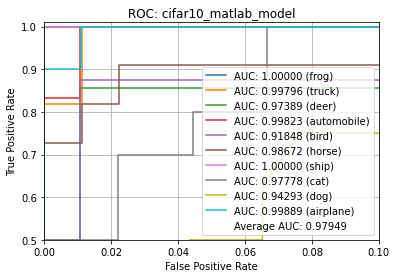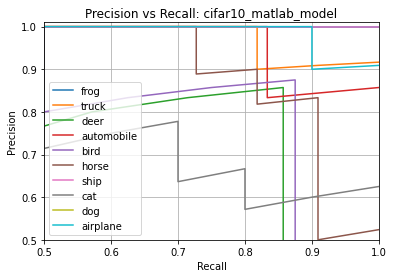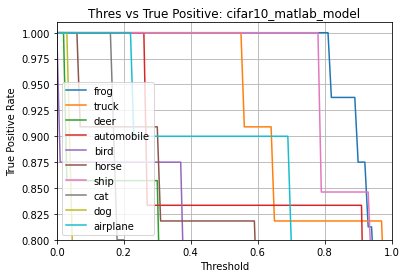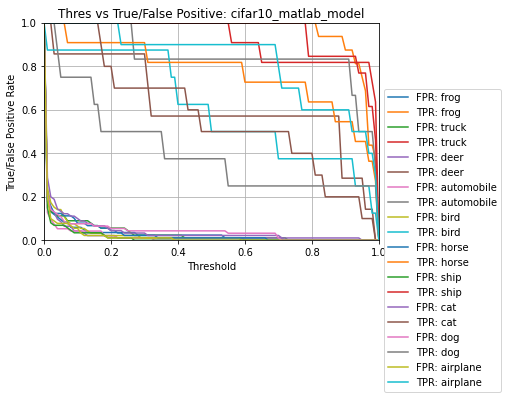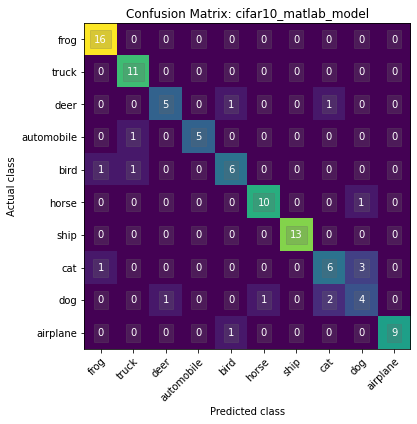## Next Steps¶

Now that we have an int8 `.tflite` model file, we can deploy it to our embedded target using the Gecko SDK.

Refer to the following links for more details: